# Solutions to SQA examination

### Paper II Solutions

```1.a.i.	Component of the force acting parallel to the slope(Fs) is calculated using:
Fs = mgsinq
Fs = 2.0x9.8xsin20o
Fs = 6.7N

a.ii.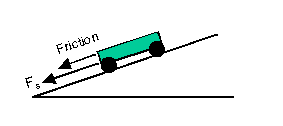a.iii.To calculate the acceleration use [N2]
a = Funbalanced/m

Funbalanced = FFriction+Fs
Funbalanced = -1.3 + (-6.7)	Where vectors acting down the slope are taken as negative.
Funbalanced = -8N

a = -8/2.0
a = -4m/s2
Which equates to a deceleration of 4m/s2, as required.

a.iv.	At its furthest point up the slope the trolley will be at rest.

v = 0m/s			v = u + at
u = 3m/s			t = (v-u)/a
a = -4m/s2			t = (0-3)/-4
t = ?				t = 0.75s

a.v.	v2 = u2 + 2as
s = (v2 - u2)/2a
s = (02 - 32)/(2x-4)
s = -9/-8
s = 1.125m

b.i.b.ii.	The force vectors in the above diagram are acting in opposite directions. This will
produce a smaller unbalanced force than that calculated in part a.iii. This means
that the magnitude of the acceleration will be less when the trolley moves down the
slope.

2.a.	The weight of the student is equal to the scale reading when the lift is moving
at a steady speed. This weight is used to calculate the mass of the student.

w = mg
m = w/g
m = 588/9.8
m = 60kg

b.	The boy is accelerating at the same rate as the lift. The unbalanced force producing
this acceleration is equal to the scale reading when accelerating minus the steady
state scale reading. The diagram below illustrates this with the scale reading
represented by the the upward force labelled T and downward force, weight,
represented by W.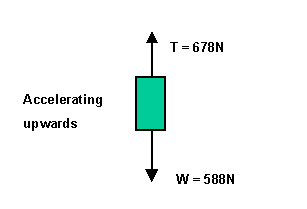Funbalanced = 678-588
Funbalanced = 90N

a = Funbalanced/m
a = 90/60
a = 1.5m/s2

c.	The lift is decelerating when the upward force is less than the weight.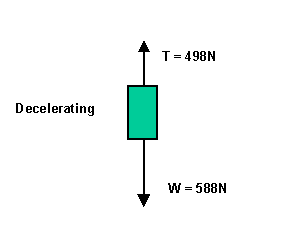Funbalanced = 498 - 588
Funbalanced = -90N		The negative indicates that the unbalanced force
acts in the downwards direction.

a = Funbalanced/m
a = -90/60
a = -1.5m/s2

This can be thought of as an upward deceleration of 1.5m/s2

d.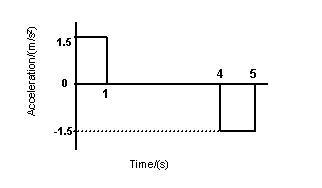3.a.i.	V = d/t1		d = diameter of ball = 24mm = 0.024m
t = time for ball to pass through light gate = 0.060s

V = 0.024/0.060
V = 0.4m/s

a.ii.	Favg = change in momentum/contact time
Favg = (mv-mu)/t
Favg = m(v-u)/t
Favg = 0.045(0.4-0)/0.005
Favg = 3.6N

b.i.	Percentage error in mass 		= (0.01/45)x100 	= 0.02%
Percentage error in contact time	= (0.001/0.005)x100 	= 20%
Percentage error in 		t1	= (0.001/0.060)x100 	= 1.67%
Percentage error in ball diameter	= (1/24)x100 		= 4.17%

The greatest uncertainty is in the contact time measurement.

b.ii.	The percentage error in the result can be taken as equal to the largest
individual error.

20% of 3.6N = (20/100)x3.6 = 0.72N

Favg = (3.6 +- 0.72)N

4.a.	Initial volume of gas (V1) in the container = (8-5)litres = 3 litres = 3 x10-3m3
Density of gas (r) = 1.29kg/m3

m = rV1
m = 1.293x(3x10-3m3)
m = 3.87x10-3Kg

b.i.	Pressure before pumping (Pbefore) = 1.01x105Pa
Pressure after pumping (P1) = 3.0x105Pa

Volume of air before and after pumping(V1) is fixed at 3x10-3m3
Area(A) = 7.0x103m2

To calculate the force, after pumping, use: P1 = F/A

F = P1A
F = 3.0x105x7.0x10-3
F = 2100N

b.ii.	When the volume of the water is 2 litres, the volume of the gas in the container(V2)
is 6 litres,or 6x10-3m3. The pressure at this time is P2.

P1V1 = P2V2
P2 = P1V1/V2
P2 = 3x105x3x10-3/6x10-3
P2 = 1.5x105Pa

5.a.i.	Vtpd = emf - Ir

I = emf/(R+r)
I = 1.5/(3+0.75)
I = 1.5/3.75
I = 0.4A

Vtpd = 1.5 - 0.4x0.75
Vtpd = 1.2V

a.ii.	Vlost = Ir		or	emf = Vtpd+Vlost
Vlost = 0.4x0.75		Vlost = emf - Vtpd
Vlost = 0.3V		 	Vlost = 1.5 - 1.2 = 0.3V

b.i.	R = 1W
Vlost = 2V

Vtpd = emf - Vlost
Vtpd = 6 - 2
Vtpd = 4V

b.ii.	I = Vtpd/Rvariable
I = 4/1
I = 4A

Vlost = Ir		Vlost = emf - Vtpd
r = Vlost/I		Vlost = 6 - 4 = 2V
r = 2/4
r = 0.5W

6.a.	A capacitor of value 5mF will store 5 coulombs of charge
per volt across it.

b.i.	Initially all the supply voltage is across the resistor in the circuit.
The current in the circuit at this point(Iinitial) is 30mA, as read
from the graph.

Iinitial = 30mA = 30x10-6A
V = 6V
R = ?

R = V/I
R = 6/30x10-6
R = 2x105W

b.ii.	Halving the value of the resistor will double the initial charging current. Lowering
the resistance will also decrease the charging time.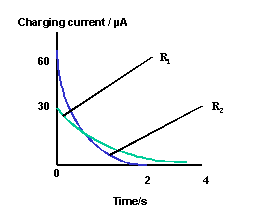c.i.	The resistance of the variable resistor must be decreased to keep the charging current
constant.

c.ii.	To calculate the total charge transferred use: Q = It
The values for I and t are read from the graph.

Q = ?
I = 100mA = 100x10-6A
t = 10s

Q = Ixt
Q = 100x10-6x10
Q = 100x10-5C = 1x10-3C = 1mC

c.iii.To calculate the capacitance use: Q = CVcapacitor
At 10s Vcapacitor = 5V

Q = 1x10-3C
Vcapacitor = 5V
C = ?

C = Q/Vcapacitor
C = 1x10-3/5
C = 2x10-4F = 200mF

7.a.i.	To calculate the kinetic energy(Ek) use : Ek = mv2/2

Ek = ?
me = 9.11x10-31kg
v = 4.2x107m/s

Ek = mv2/2
Ek = 9.11x10-31x(4.2x107)2/2
Ek = 8.03x10-16J

7.a.ii.	The gain in the kinetic energy of the electron, with charge e, is a result of it being
accelerated by a potential difference V.

Ek(gain) = eV
V = Ek(gain)/e
V = 8.03x10-16/1.6x10-19
V = 5021.9V

b.	Plate P can be made more positive to attract the electron. This will move the
spot up vertically towards X.
Making Q more positive will attract the electron and move it horizontally
towards X.If plate Q is made twice as positive as plate P the combined effect
will be to move the spot to position X.

8.a.i.	The amplifier is working in inverting mode.

a.ii.	Gain =  -Rfeedback/Rinput

Rfeedback = 2x106W
Rinput = 100x103W

Gain = -2x106/100x103
Gain = -20

a.iii.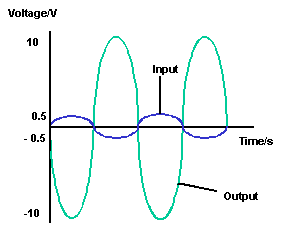b.i.	Between 2ms and 8ms the gain of the amplifier is said to be saturated. This is due to
the fact that the output voltage from the amplifier cannot be greater than that supplied
to it.

b.ii.	To produce saturation you can:increase the size of the feedback resistor;decrease the size of the input resistor;increase the size of the input voltage.

9.a.	DE32 = E3-E2
DE32 = -2.416x10-19-(-5.524x10-19)
DE32 = 3.006x10-19J

To calculate the wavelength of a photon of this energy use : E = hf = hc/l

l = hc/E32
l = 6.63x10-34x3x108/3.006x10-19
l = 6.617x10-7m = 661.7nm

This corresponds to spectral line Z.

This answer can also be deduced by the fact that the photon produced by the transition
between the energy levels E3 and E2has the lowest energy of the four in the diagram.
This lowest energy photon will also have the longest wavelength,which is Z.

b.	The more photons of a particular frequency emitted the brighter the spectral line
will be. This means that there must be more electron transitions between the energy
levels producing the lines Y and Z.

c.i.  E = hf
E = 6.63x10-34x7.486x1013
E = 4.96x10-20J

c.ii.	DE54 = E5-E4
DE54 = -0.864x10-19-(-1.360x10-19)
DE54 = 4.96X10-20J

The transition between energy levels E5 and E4 produces the infrared photon.

10.a.i.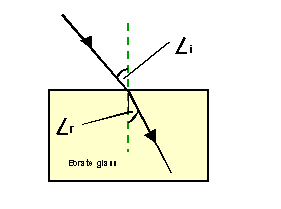Angle i = 50o
Angle r = 28o

nglass = sin(i)/sin(r)
nglass = sin 50o/sin 28o
nglass = 0.766/0.496
nglass = 1.63

a.ii.	qcritical = sin-1(1/n)
qcritical = sin-1(1/1.63)
qcritical = sin-1(0.613)
qcritical = 37.8o

b.i.	nquartz = lair/lquartz
lquartz = lair/nquartz
lquartz = 510/1.55
lquartz = 329nm

b.ii.	As the wavelength of visible light increases the refractive index of the quartz(nquartz)
decreases. This means that (1/nquartz) increases and therefore qcritical[sin-1(1/nquartz)]
also increases.

b.iii.Flint glass has a higher refractive index than crown glass for all wavelengths of light.
This would result in the spectrum being projected onto a lower position on the screen.
Additionally, because the difference in refractive index across the spectrum is greater
with flint glass, the distance between the red end and the violet end of the spectrum,
as appearing on the screen, would be greater.

11.a.i.	To conserve atomic and mass number in the decay of Thorium to Palladium a
beta particle(b, 0-1e)is emitted.

a.ii.	Number of neutrons(n) = mass number(A) - atomic number(Z)
n = 238 - 92
n = 146

a.iii.23492U -> 23090Th + 42He

b.i.	Soil and rocks containing natural radioactive isotopes, and cosmic rays,  contribute

b.ii.	The earths atmosphere absorbs cosmic radiation and reduces the effective dose equivalent
rate at sea level. At heights above 4km there is less atmosphere to absorb the radiation
which results in a higher dose equivalent rate at these rarefied altitudes.

b.iii.
(A)t = 7h
H/t = 5mSv/h
H = ?

H = tx5mSv/h
H = 7hx5mSv/h
H(flight) = 35mSv

(B)During 40 flights: H = 40x35mSv

H = 1400mSv (As a result of flights)

In addition to this the traveller will be exposed to sea level background radiation.
Time at sea level tsea = (365x24)-(7x40) = 8480h
H(at sea level) = 8480hx0.2mSv/h
H(at sea level) = 1696mSv

H(total) = H(at sea level) + H(flight)
H(total) = 1696mSv + 1400mSv
H(total) = 3096mSv = 3.096(mSv/year)

END OF PAPER